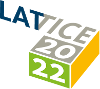#The 39th International Symposium on Lattice Field Theory (Lattice 2022)

Aug 8 – 13, 2022
Hörsaalzentrum Poppelsdorf
Europe/Berlin timezone

## Infinite Variance in Fermionic Systems

Aug 11, 2022, 9:00 AM
20m
CP1-HSZ/1.004 (CP1-HSZ) - HS7 (CP1-HSZ)

### CP1-HSZ/1.004 (CP1-HSZ) - HS7

#### CP1-HSZ

70
Show room on map
Oral Presentation Algorithms (including Machine Learning, Quantum Computing, Tensor Networks)

### Speaker

Cagin Yunus (MIT)

### Description

In Monte Carlo simulations of lattice quantum field theories, if the variance of an estimator of a particular quantity is formally infinite, or very large compared to the square of the mean, then expectation of the estimator can not be reliably obtained using the given sampling procedure. A particularly simple example is given by the Gross-Neveu model where Monte Carlo calculations involve the introduction of auxiliary bosonic variables through a Hubbard-Stratonovich (HS) transformation. Here, it is shown that the variances of HS estimators for classes of operators involving fermion fields are divergent in this model. To correctly estimate these observables, an infinite sequence of discrete Hubbard-Stratonovich transformations and a reweighting procedure that can be applied to any non-negative observable are introduced.

### Primary authors

Cagin Yunus (MIT) William Detmold (MIT)